• Last Updated : 17 Oct, 2021

To add enemy to our game we are going to follow the below steps:

• First, we will create a variable to store the enemy sprite.

Syntax:

` self.enemy = None`
• After that, we will create one more variable to store the velocity of the enemy.

Syntax:

`self.enemy_move = velocity`
• Store our enemy sprite in the enemy variable.

Syntax:

`self.enemy = arcade.Sprite("path",1)`
• Set the initial coordinates.

Syntax:

```self.enemy.center_x = value
self.enemy.center_y = value```
• Add the enemy sprite in the scene.

Syntax:

`self.scene.add_sprite("Enemy", self.enemy)`
• Move the enemy by changing the x coordinate.

Syntax:

`self.enemy.center_x += self.enemy_move`
• Check for collisions between the player and enemy.

Syntax:

self.player_sprite, self.scene.get_sprite_list(“Enemy”)

)

• If they are colliding then remove the player sprite.

Syntax:

for enemy in enemy_collide:

self.player_sprite.remove_from_sprite_lists()

## Functions Used:

• draw_text(): This function is used to draw text to the screen using Pyglet’s label.

Syntax: arcade.draw_text(text, x, y, color, size, width, align, font_name)

Parameters:

text: Text we want to display

x : x coordinate

y : y coordinate

color : color of the text

size : Size of the font

width : Width of the text

align : Alignment of the text

font_name : Name of the font

• Camera(): The Camera class is used for controlling the visible viewport.

Syntax: arcade.Camera( width , height, window)

Parameters:

width: width of the viewport

height: height of the viewport

window: Window to associate with this camera

• Scene(): A class that represents a scene object.

Parameters:

sprite_lists: A list of SpriteList objects

name_mapping: A dictionary of SpriteList objects

• PhysicsEnginePlatformer(): Simplistic physics engine, used for platform.

Parameters:

player_sprite: sprite of the player

platforms: The sprites it can’t move through

gravity: Downward acceleration per frame

## Sprites Used: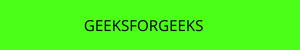PLATFORM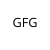PLAYER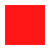ENEMY

In the below example, we are going to create a MainGame() class. Inside this class first, we are going to initialize some variables for velocity, camera, score, level, enemy, enemy sprite, and player’s sprite then we will create 6 functions inside this class.

• on_draw(): Inside this function, we will use our camera and draw the scene and our score text on the screen.
• setup(): In this function, we will initialize our camera and scene object then we will load our player and platform’s sprites then we will load our enemy sprite and initialize the starting x and y coordinate. After that, we will call the PhysicsEnginePlatformer() function.
• on_update(): In this function, we will update the x coordinates of the player’s sprite, camera, and physics engine. We will also add our enemy_move variable in the x coordinates of the enemy sprite. Then we will check the collision between the enemy and the player. And if the player is colliding with the enemy then we will remove the player sprite.
• on_key_press() and on_key_release(): In this function, we will change the value of the velocity variable according to the keyboard key that is pressed or released.
• camera_move(): In this function, we will move our camera according to our player’s current position.

## Python3

 `# Importing arcade module``import` `arcade`` ` `# Creating MainGame class``class` `MainGame(arcade.Window):``    ``def` `__init__(``self``):``        ``super``().__init__(``600``, ``600``,``                         ``title``=``"Player Movement"``)`` ` `        ``# Initializing a variable to store``        ``# the velocity of the player``        ``self``.vel_x ``=` `0`` ` `        ``# Creating variable for Camera``        ``self``.camera ``=` `None`` ` `        ``# Creating variable to store current score``        ``self``.score ``=` `0`` ` `        ``# Creating variable to move the enemy``        ``self``.enemy_move ``=` `5`` ` `        ``# Creating variable for enemy sprite``        ``self``.enemy ``=` `None`` ` `        ``# Creating scene object``        ``self``.scene ``=` `None`` ` `        ``# Creating variable to store player sprite``        ``self``.player ``=` `None`` ` `        ``# Creating variable for our game engine``        ``self``.physics_engine ``=` `None`` ` `    ``# Creating on_draw() function to draw on the screen``    ``def` `on_draw(``self``):``        ``arcade.start_render()`` ` `        ``# Drawing the text``        ``arcade.draw_text(``'Score :- '``+``str``(``self``.score), ``                         ``self``.player_sprite.center_x ``-` `500` `/` `2``,``                         ``self``.player_sprite.center_y ``+` `200``,``                         ``arcade.color.WHITE, ``30``, ``5000``, ``'left'``)`` ` `        ``# Using the camera``        ``self``.camera.use()``         ` `        ``# Drawing our scene``        ``self``.scene.draw()`` ` `    ``def` `setup(``self``):``         ``# Initialize Scene object``        ``self``.scene ``=` `arcade.Scene()``         ` `        ``# Using Camera() function``        ``self``.camera ``=` `arcade.Camera(``600``, ``600``)`` ` `        ``# Loading enemy sprite``        ``self``.enemy ``=` `arcade.Sprite(``"Enemy.png"``, ``1``)`` ` `        ``# Initializing the starting coordinates``        ``self``.enemy.center_x ``=` `500``        ``self``.enemy.center_y ``=` `110`` ` `        ``# Adding the enemy sprite in the scene``        ``self``.scene.add_sprite(``"Enemy"``, ``self``.enemy)`` ` `        ``# Creating different sprite lists``        ``self``.scene.add_sprite_list(``"Player"``)``        ``self``.scene.add_sprite_list(``"Platforms"``,``                                   ``use_spatial_hash``=``True``)`` ` `        ``# Adding player sprite``        ``self``.player_sprite ``=` `arcade.Sprite(``"Player.png"``, ``1``)`` ` `        ``# Adding coordinates for the center of the sprite``        ``self``.player_sprite.center_x ``=` `64``        ``self``.player_sprite.center_y ``=` `90`` ` `        ``# Adding Sprite in our scene``        ``self``.scene.add_sprite(``"Player"``, ``self``.player_sprite)`` ` `        ``# Adding platform sprite according to level``        ``platform ``=` `arcade.Sprite(f``"Platform.png"``, ``1``)``         ` `        ``# Adding coordinates for the center of the platform``        ``platform.center_x ``=` `300``        ``platform.center_y ``=` `32``        ``self``.scene.add_sprite(``"Platforms"``, platform)`` ` `        ``# Creating Physics engine``        ``self``.physics_engine ``=` `arcade.PhysicsEnginePlatformer(``            ``self``.player_sprite, ``self``.scene.get_sprite_list(``"Platforms"``), ``0.5``        ``)`` ` `    ``# Creating on_update function to``    ``# update the x coordinate``    ``def` `on_update(``self``, delta_time):`` ` `        ``# Moving the position of enemy``        ``self``.enemy.center_x ``+``=` `self``.enemy_move`` ` `        ``# Changing the direction of enemy movement``        ``if` `self``.enemy.center_x > ``500` `or` `self``.enemy.center_x < ``160``:``            ``self``.enemy_move ``*``=` `-``1`` ` `        ``# Checking if the enemy is colliding with player or not``        ``enemy_collide ``=` `arcade.check_for_collision_with_list(``            ``self``.player_sprite, ``self``.scene.get_sprite_list(``"Enemy"``)``        ``)`` ` `        ``# Looping through each enemy and removing the player``        ``# if colliding``        ``for` `enemy ``in` `enemy_collide:``            ``# Remove the coin``            ``self``.player_sprite.remove_from_sprite_lists()`` ` `        ``# Changing x coordinate of player``        ``self``.player_sprite.center_x ``+``=` `self``.vel_x ``*` `delta_time``         ` `        ``# Updating the physics engine to move the player``        ``self``.physics_engine.update()`` ` `        ``# Calling the camera_move function``        ``self``.camera_move()`` ` `    ``# Creating function to change the velocity``    ``# when button is pressed``    ``def` `on_key_press(``self``, symbol, modifier):`` ` `        ``# Checking the button pressed``        ``# and changing the value of velocity``        ``if` `symbol ``=``=` `arcade.key.LEFT:``            ``self``.vel_x ``=` `-``300``        ``elif` `symbol ``=``=` `arcade.key.RIGHT:``            ``self``.vel_x ``=` `300``        ``elif` `symbol ``=``=` `arcade.key.UP:``            ``if` `self``.physics_engine.can_jump():``                ``self``.player_sprite.change_y ``=` `15`` ` `    ``# Creating function to change the velocity``    ``# when button is released``    ``def` `on_key_release(``self``, symbol, modifier):`` ` `        ``# Checking the button released``        ``# and changing the value of velocity``        ``if` `symbol ``=``=` `arcade.key.LEFT:``            ``self``.vel_x ``=` `0``        ``elif` `symbol ``=``=` `arcade.key.RIGHT:``            ``self``.vel_x ``=` `0`` ` `    ``def` `camera_move(``self``):`` ` `        ``# Getting the x coordinate for the center of camera``        ``screen_x ``=` `self``.player_sprite.center_x ``-` `\``            ``(``self``.camera.viewport_width ``/` `2``)`` ` `        ``# Getting the y coordinate for the center of camera``        ``screen_y ``=` `self``.player_sprite.center_y ``-` `\``            ``(``self``.camera.viewport_height ``/` `2``)`` ` `        ``# Moving the camera``        ``self``.camera.move_to([screen_x, screen_y])`` ` ` ` `# Calling MainGame class``game ``=` `MainGame()``game.setup()``arcade.run()`

Output: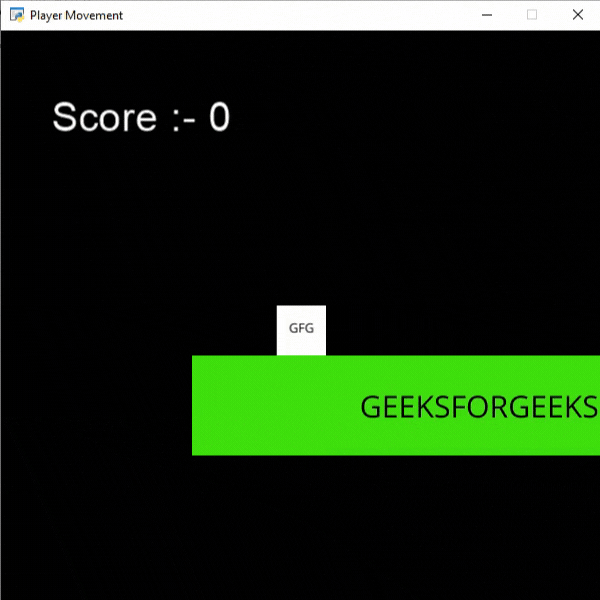## Killing the Enemy

Now we want to kill the enemy when the player jumps on top of it. For this, we will check if the bottom other player is equal to the top of the enemy or not. If it is equal then we will remove the layer sprite.

• For this first, we will store the coordinate of the bottom of the player in a variable.

Syntax:

x = int(self.player_sprite.center_y – 14)

• Similarly the coordinate of the top of the enemy in a different variable.

Syntax:

y = int(self.enemy.center_y + 25)

• Then, if x and y are equal and the enemy and player are colliding then we will remove our enemy sprite.

Syntax:

if x == y:

self.enemy.remove_from_sprite_lists()

## Python3

 `# Importing arcade module``import` `arcade`` ` `# Creating MainGame class``class` `MainGame(arcade.Window):``    ``def` `__init__(``self``):``        ``super``().__init__(``600``, ``600``,``                         ``title``=``"Player Movement"``)`` ` `        ``# Initializing a variable to store``        ``# the velocity of the player``        ``self``.vel_x ``=` `0`` ` `        ``# Creating variable for Camera``        ``self``.camera ``=` `None`` ` `        ``# Creating variable to store current score``        ``self``.score ``=` `0`` ` `        ``# Creating variable to move the enemy``        ``self``.enemy_move ``=` `2`` ` `        ``# Creating variable for enemy sprite``        ``self``.enemy ``=` `None`` ` `        ``# Creating scene object``        ``self``.scene ``=` `None`` ` `        ``# Creating variable to store player sprite``        ``self``.player ``=` `None`` ` `        ``# Creating variable for our game engine``        ``self``.physics_engine ``=` `None`` ` `    ``# Creating on_draw() function to draw on the screen``    ``def` `on_draw(``self``):``        ``arcade.start_render()`` ` `        ``# Drawing the text``        ``arcade.draw_text(``'Score :- '``+``str``(``self``.score), ``                         ``self``.player_sprite.center_x ``-` `500` `/` `2``,``                         ``self``.player_sprite.center_y ``+` `200``,``                         ``arcade.color.WHITE, ``30``, ``5000``, ``'left'``)`` ` `        ``# Using the camera``        ``self``.camera.use()``         ` `        ``# Drawing our scene``        ``self``.scene.draw()`` ` `    ``def` `setup(``self``):``       ` `         ``# Initialize Scene object``        ``self``.scene ``=` `arcade.Scene()``         ` `        ``# Using Camera() function``        ``self``.camera ``=` `arcade.Camera(``600``, ``600``)`` ` `        ``# Loading enemy sprite``        ``self``.enemy ``=` `arcade.Sprite(``"Enemy.png"``, ``1``)`` ` `        ``# Initializing the starting coordinates``        ``self``.enemy.center_x ``=` `500``        ``self``.enemy.center_y ``=` `110`` ` `        ``# Adding the enemy sprite in the scene``        ``self``.scene.add_sprite(``"Enemy"``, ``self``.enemy)`` ` `        ``# Creating different sprite lists``        ``self``.scene.add_sprite_list(``"Player"``)``        ``self``.scene.add_sprite_list(``"Platforms"``,``                                   ``use_spatial_hash``=``True``)`` ` `        ``# Adding player sprite``        ``self``.player_sprite ``=` `arcade.Sprite(``"Player.png"``, ``1``)`` ` `        ``# Adding coordinates for the center of the sprite``        ``self``.player_sprite.center_x ``=` `64``        ``self``.player_sprite.center_y ``=` `90`` ` `        ``# Adding Sprite in our scene``        ``self``.scene.add_sprite(``"Player"``, ``self``.player_sprite)`` ` `        ``# Adding platform sprite according to level``        ``platform ``=` `arcade.Sprite(f``"Platform.png"``, ``1``)``         ` `        ``# Adding coordinates for the center of the platform``        ``platform.center_x ``=` `300``        ``platform.center_y ``=` `32``        ``self``.scene.add_sprite(``"Platforms"``, platform)`` ` `        ``# Creating Physics engine``        ``self``.physics_engine ``=` `arcade.PhysicsEnginePlatformer(``            ``self``.player_sprite, ``self``.scene.get_sprite_list(``"Platforms"``), ``0.5``        ``)`` ` `    ``# Creating on_update function to``    ``# update the x coordinate`` ` `    ``def` `on_update(``self``, delta_time):`` ` `        ``# Moving the position of enemy``        ``self``.enemy.center_x ``+``=` `self``.enemy_move`` ` `        ``# Changing the direction of enemy movement``        ``if` `self``.enemy.center_x > ``500` `or` `self``.enemy.center_x < ``160``:``            ``self``.enemy_move ``*``=` `-``1`` ` `        ``# Checking if the enemy is colliding with player or not``        ``enemy_collide ``=` `arcade.check_for_collision_with_list(``            ``self``.player_sprite, ``self``.scene.get_sprite_list(``"Enemy"``)``        ``)`` ` `        ``# Looping through each enemy and removing the player if colliding``        ``for` `enemy ``in` `enemy_collide:`` ` `            ``# Checking the top of enemy and bottom of player``            ``x ``=` `int``(``self``.player_sprite.center_y ``-` `14``)``            ``y ``=` `int``(``self``.enemy.center_y ``+` `25``)``            ``if` `x ``=``=` `y:``                ``self``.enemy.remove_from_sprite_lists()``            ``else``:``                ``# Remove the coin``                ``self``.player_sprite.remove_from_sprite_lists()`` ` `        ``# Changing x coordinate of player``        ``self``.player_sprite.center_x ``+``=` `self``.vel_x ``*` `delta_time``         ` `        ``# Updating the physics engine to move the player``        ``self``.physics_engine.update()`` ` `        ``# Calling the camera_move function``        ``self``.camera_move()`` ` `    ``# Creating function to change the velocity``    ``# when button is pressed``    ``def` `on_key_press(``self``, symbol, modifier):`` ` `        ``# Checking the button pressed``        ``# and changing the value of velocity``        ``if` `symbol ``=``=` `arcade.key.LEFT:``            ``self``.vel_x ``=` `-``300``        ``elif` `symbol ``=``=` `arcade.key.RIGHT:``            ``self``.vel_x ``=` `300``        ``elif` `symbol ``=``=` `arcade.key.UP:``            ``if` `self``.physics_engine.can_jump():``                ``self``.player_sprite.change_y ``=` `15`` ` `    ``# Creating function to change the velocity``    ``# when button is released``    ``def` `on_key_release(``self``, symbol, modifier):`` ` `        ``# Checking the button released``        ``# and changing the value of velocity``        ``if` `symbol ``=``=` `arcade.key.LEFT:``            ``self``.vel_x ``=` `0``        ``elif` `symbol ``=``=` `arcade.key.RIGHT:``            ``self``.vel_x ``=` `0`` ` `    ``def` `camera_move(``self``):`` ` `        ``# Getting the x coordinate for the center of camera``        ``screen_x ``=` `self``.player_sprite.center_x ``-` `\``            ``(``self``.camera.viewport_width ``/` `2``)`` ` `        ``# Getting the y coordinate for the center of camera``        ``screen_y ``=` `self``.player_sprite.center_y ``-` `\``            ``(``self``.camera.viewport_height ``/` `2``)`` ` `        ``# Moving the camera``        ``self``.camera.move_to([screen_x, screen_y])`` ` ` ` `# Calling MainGame class``game ``=` `MainGame()``game.setup()``arcade.run()`

Output: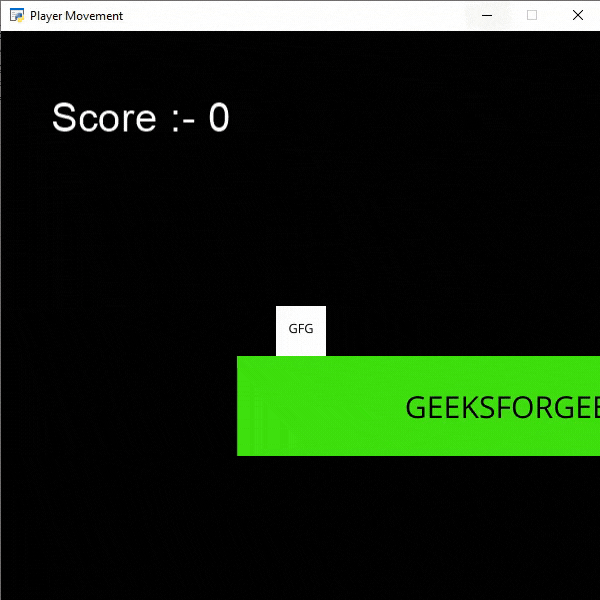My Personal Notes arrow_drop_up